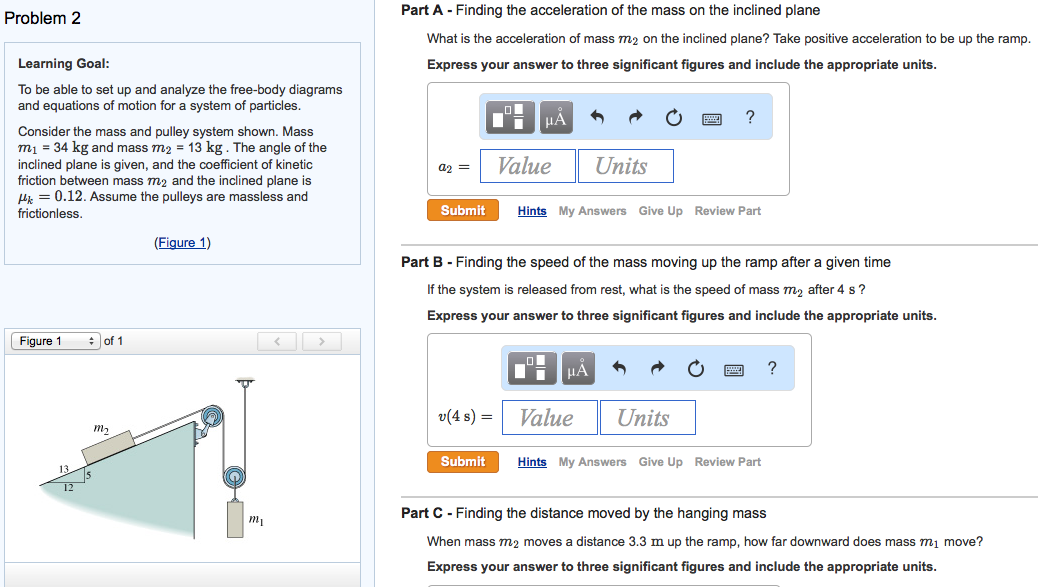### Create an Account

Home / Questions / Image for Problem 2 Learning Goal To be able to set up and analyze the free-body diagrams ...

# Image for Problem 2 Learning Goal To be able to set up and analyze the free-body diagrams and equations of motion for a Show transcribed image text Problem 2 Learning Goal To be able to set up andShow transcribed image text Problem 2 Learning Goal: To be able to set up and analyze the free-body diagrams and equations of motion for a system of particles. Consider the mass and pulley system shown. Mass m1 =34kg and mass m2 = 13 kg. The angle of the inclined plane is given, and the coefficient of kinetic friction between mass m2 and the inclined plane is muk = 0.12. Assume the pulleys are massless and frictionless. Part A - Finding the acceleration of the mass on the inclined plane What is the acceleration of mass m2 on the inclined plane? Take positive acceleration to be up the ramp. Express your answer to three significant figures and include the appropriate units. Part B - Finding the speed of the mass moving up the ramp after a given time if the system is released from rest, what is the speed of mass m2 after 4 s? Express your answer to three significant figures and include the appropriate units. Part C - Finding the distance moved by the hanging mass When mass m2 moves a distance 3.3 m up the ramp, how far downward does mass m1 move? Express your answer to three significant figures and include the appropriate units.

May 19 2020 View more View LessSubscribe To Get Solution